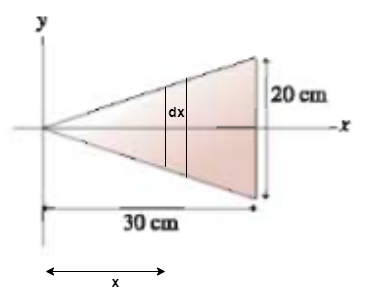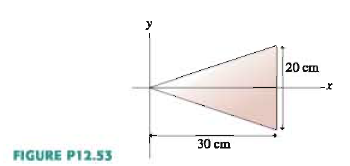# Problem: An 800 g steel plate has the shape of the isosceles triangle shown in figure P12.53. What are the x-and y- coordinates of the center of mass?

###### FREE Expert Solution

The first thing is to divide the triangle into vertical strips of width dx and mass dm. Let's consider one strip, whose center is at a distance x-from the origin.Area of the triangle is given by:

A = (1/2)bh = (1/2)(20 × 10-2)(30 × 102) = 0.03 m2

Let the length of the small strip be l.

The area of this strip is expressed as:

dA = l·dx

The mass of the strip/the element is:

dm = (M/A)dA = (800 × 10-3/0.03)ldx = 26.67 l·dx kg/m2

From proteries of similar triangles:

l/20 = x/30

l = (20/30)x = (2/3)x

Substituting to the expression of dm:

dm = (26.67)(2/3)x dx

91% (210 ratings)###### Problem Details

An 800 g steel plate has the shape of the isosceles triangle shown in figure P12.53. What are the x-and y- coordinates of the center of mass?